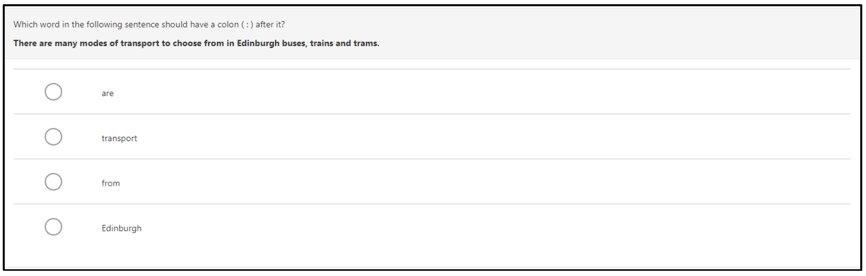Publication - Publication

# Scottish National Standardised Assessments: national report

Published: 31 Jan 2020
From:
Deputy First Minister, +1 more …
Directorate:
Learning Directorate
Part of:
Education
ISBN:
9781839605277

Summary of outcomes at a national level on the Scottish National Standardised Assessments (SNSA) in the 2018 to 2019 academic year.

114 page PDF

6.7 MB

114 page PDF

6.7 MB

Contents
Scottish National Standardised Assessments: national report

114 page PDF

6.7 MB

### Appendix 3: Additional example items

5.3.1 Example numeracy items

Number

Figure A1: Example of a P1 Number question: assessing ability to continue a counting pattern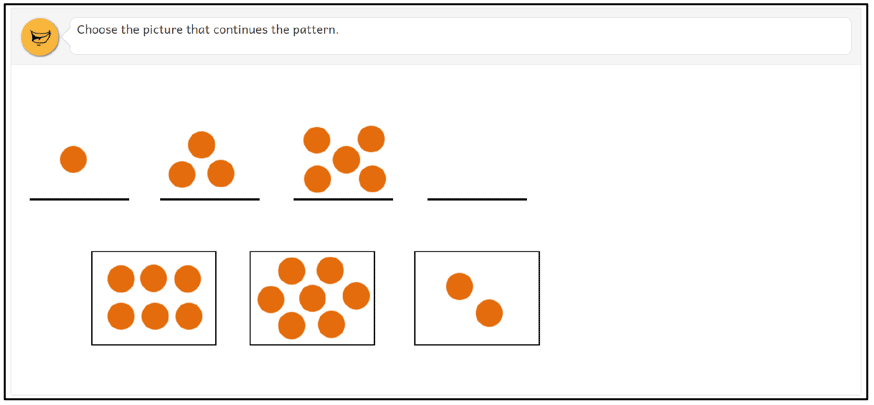Figure A2: Example of a P4 Number question: assessing ability to understand terminology associated with multiplication to complete a calculation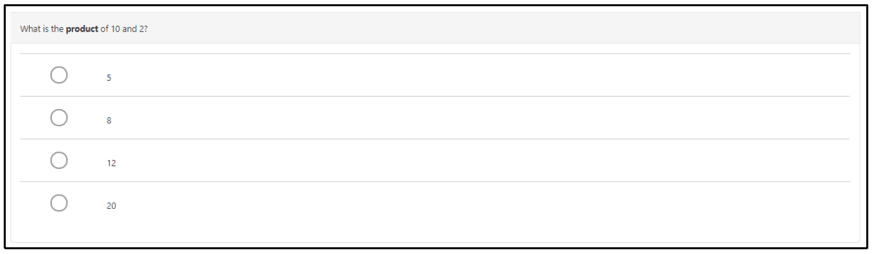Fractions, decimal fractions and percentages

Figure A3: Example of a P4 Fractions, decimal fractions and percentages question: assessing ability to compare and order fractions by size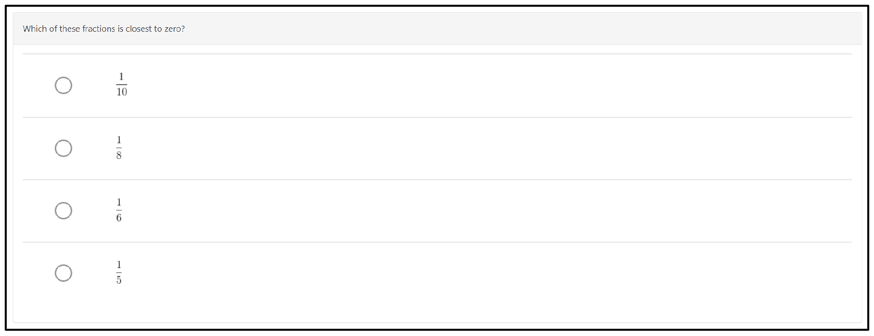Figure A4: Example of P7 Fractions, decimal fractions and percentages question: assessing ability to find a fraction of a quantity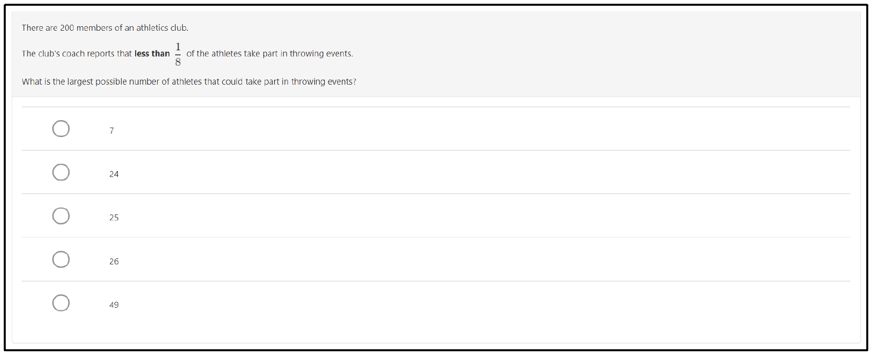Measurement, time and money

Figure A5: Example of a P1 Measurement, time and money question: assessing ability to order objects by height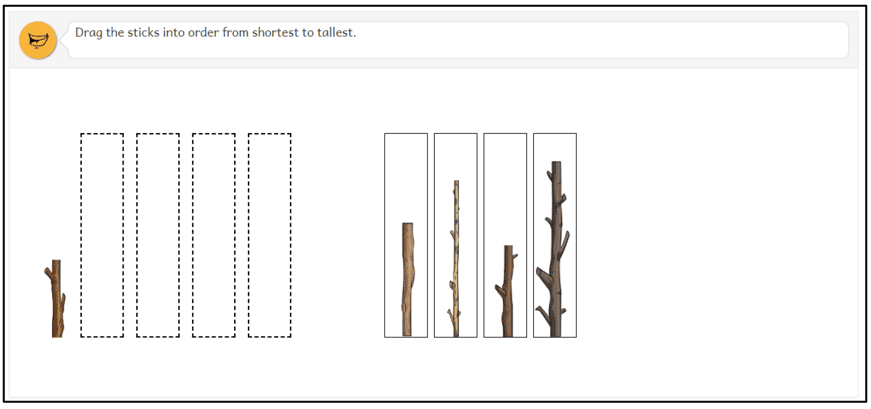Figure A6: Example of a P7 Measurement, time and money question: assessing ability to compare items to determine best value for money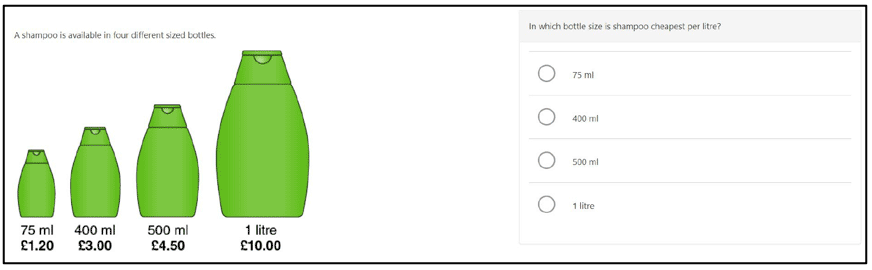Information handling

Figure A7: Example of a P1 Information handling question: assessing ability to use a chart to sort objects by a given criterion (shape)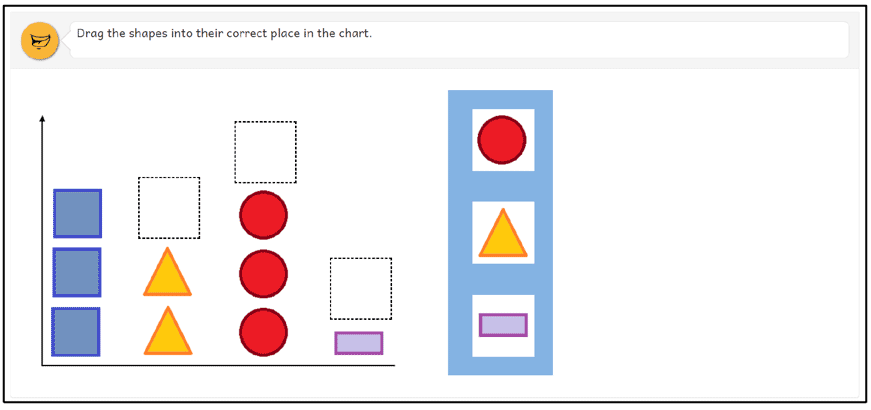Figure A8: Example of an S3 Information handling question: assessing ability to interpret a pie chart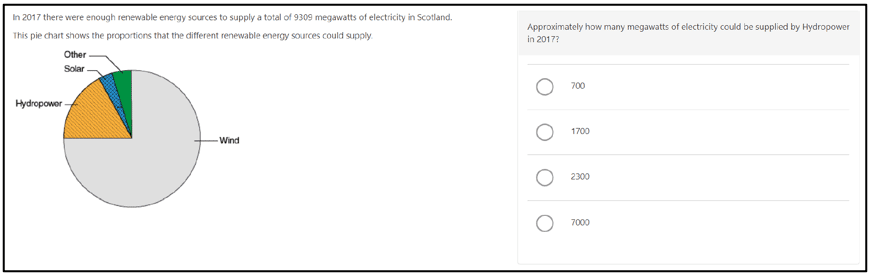Figure A9: Example of a P1 Tools for reading question: 'Select letter sound t'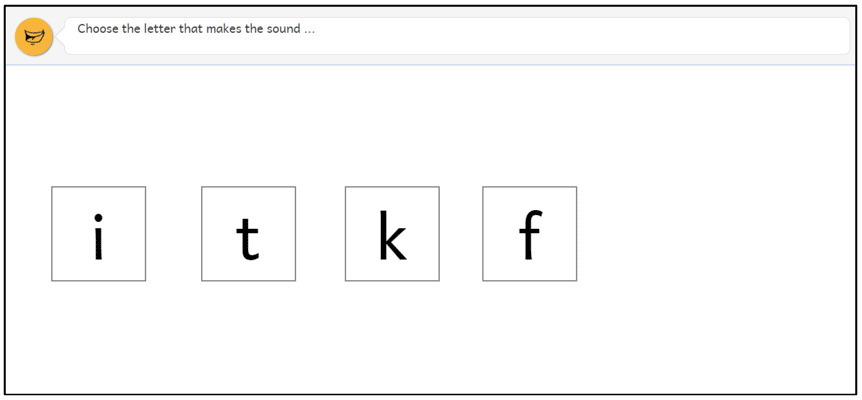Figure A10: Example of a P1 Tools for reading question: 'Match word to picture (stick)'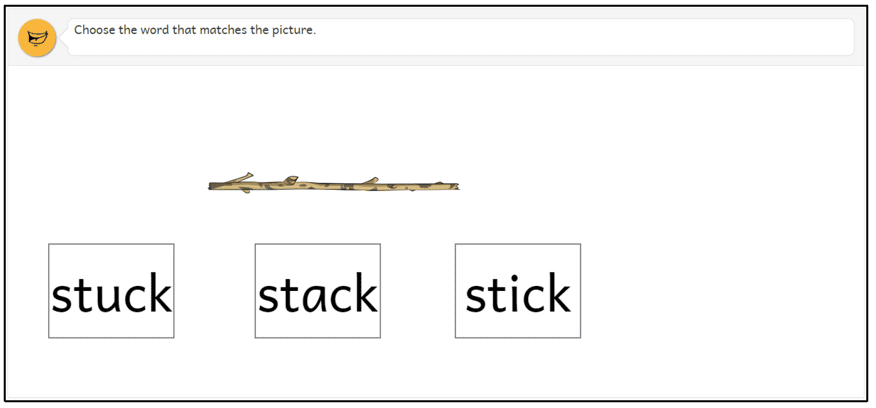Finding and using information

Figure A11: Example of a P4 reading text with a Finding and using information question: 'Cawky Question 2'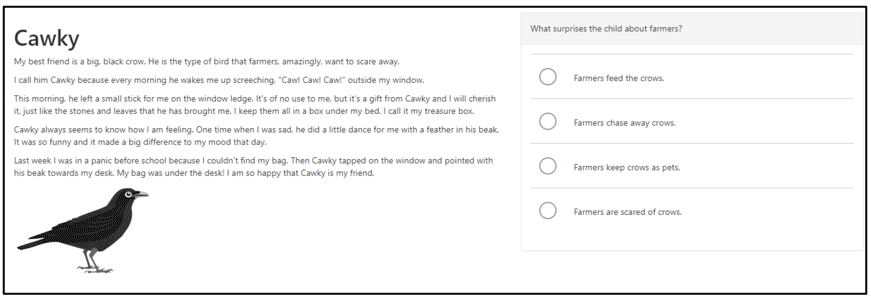Understanding, analysing and evaluating

Figure A12: Example of a P4 reading text with an Understanding, analysing and evaluating question: 'Cawky Question 3'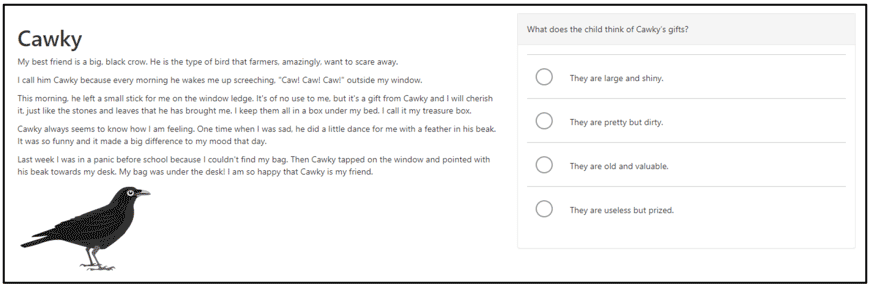Figure A13: Example of a P7 Understanding, analysing and evaluating question: 'Aphids Question 2'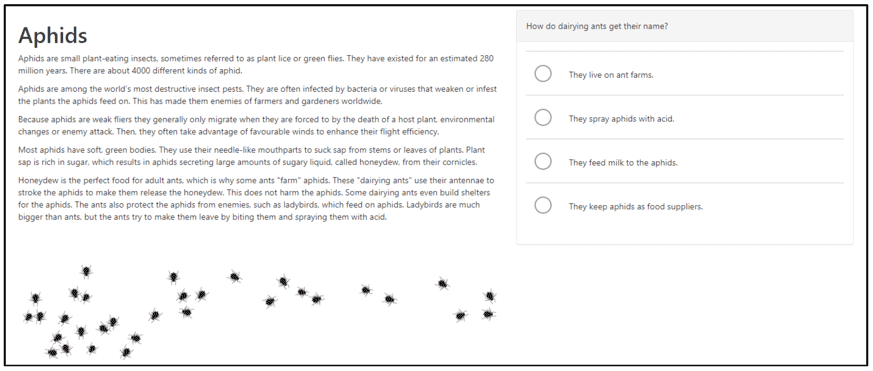Figure A14: Example of an S3 Understanding, analysing and evaluating question: 'Shill Reviewing Question 3'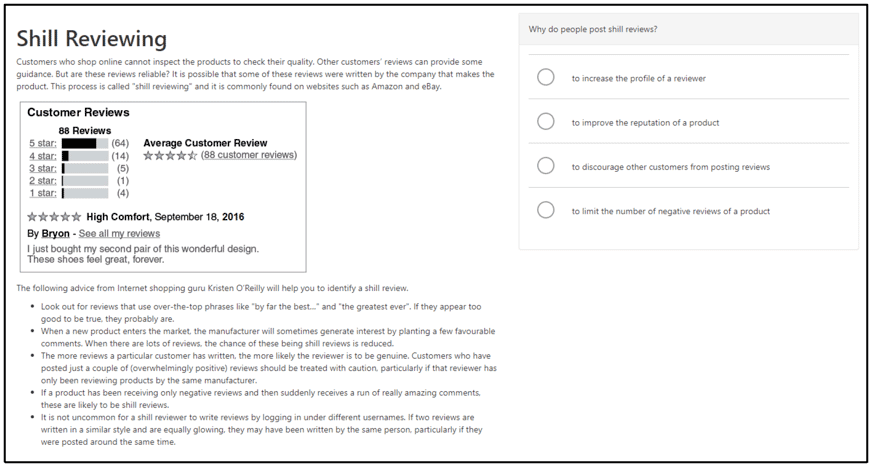Figure A15: Example of an S3 Understanding, analysing and evaluating question: 'Shill Reviewing Question 5'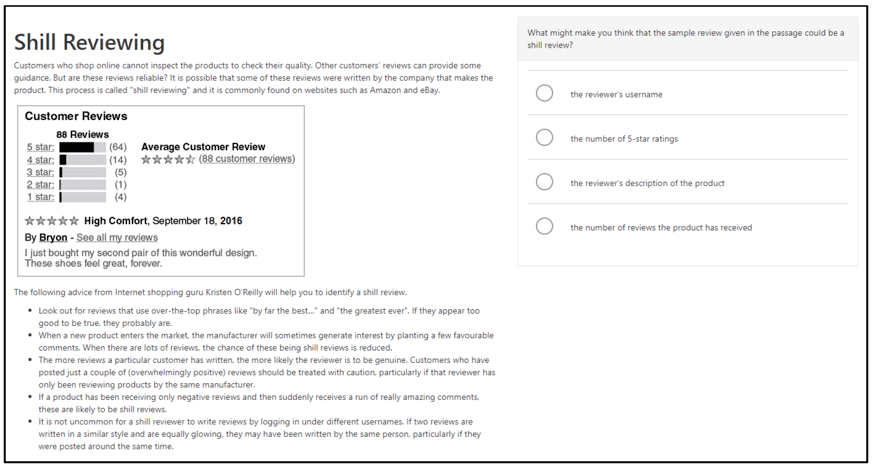5.3.3 Example writing items

Spelling

Figure A16: Example of an S3 writing unit with a Spelling question: assessing the ability to identify the correct spelling of a word with a doubled consonant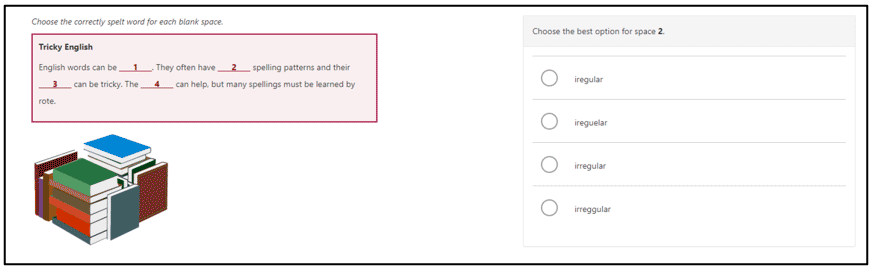Figure A17: Example of an S3 writing unit with a Spelling question: assessing the ability to identify the correct spelling of a less common word with five syllables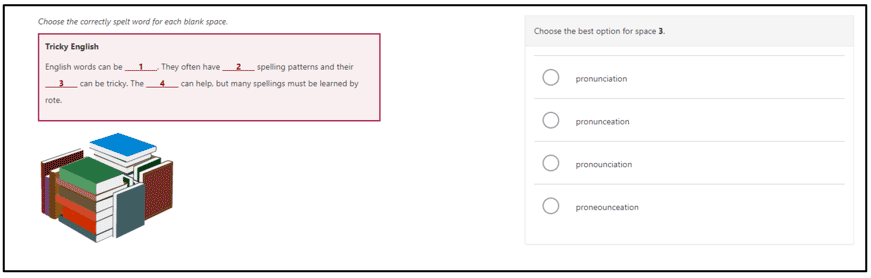Grammar

Figure A18: Example of a P4 Grammar question: assessing the ability to identify a correct pronoun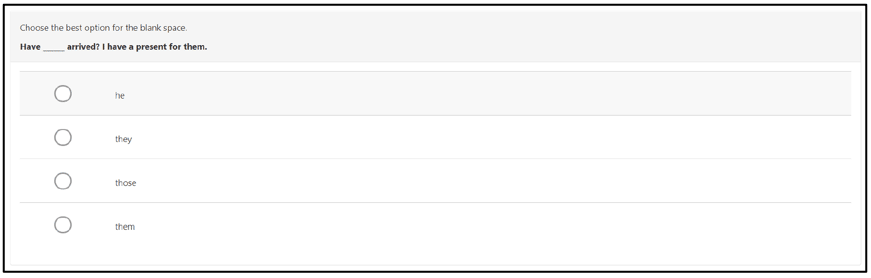Figure A19: Example of a P4 Grammar question, assessing: identify a correct verb form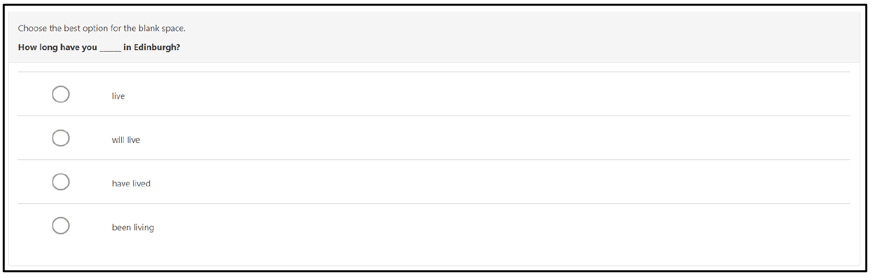Punctuation

Figure A20: Example of a P7 Punctuation question: assessing the ability to identify the correct position of a colon in a sentence